# Basic Arithmetic : Linear Equations with Ratios and Rates

## Example Questions

### Example Question #1 : Linear Equations With Ratios And Rates

I put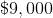in a bank account at a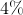annual simple interest rate. How much interest will have accumulated after two years?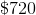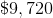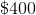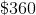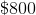Explanation:

This is a simple interest rate problem, for which we use the formula:

Interest = P x r x t

P is the principal, or original loan amount; r is the annual interest rate; and t is the number of years in question.

In this problem, P = $9,000; r = 4%; and t = 2 years. Plugging these into the formula gives us: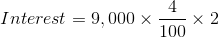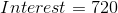So, after two years the account has gathered$720 in interest.

### Example Question #1 : Linear Equations With Ratios And Rates

Ifpainters can painthouses inday, how many painters would it take to paint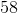houses inday?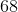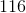Explanation:

We can set up the following proportions using the given information: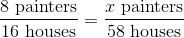Now, cross multiply these two fractions to get the following equation: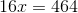Divide both sides by.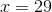### Example Question #1 : Linear Equations With Ratios And Rates

If a car travels atmiles per hour, how many hours would it take the car to travelmiles?Explanation:

We can write 40 miles per hour as the following fraction: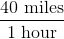Since we want to figure out how many hours it takes a car to travel 200 miles, we can write the following equation: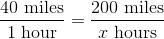Now, cross multiply.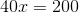Divide both sides by 40.### Example Question #4 : Linear Equations With Ratios And Rates

If one recipe calls foreggs to makewaffles, how many eggs are needed to makewaffles?Explanation:

To solve this problem, we can set up a proportion.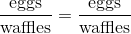That proportion will tell us how many eggs go into each waffle. Since the number of eggs that goes into each waffle doesn't change, we can set these two proportions equal to each other.

Now, fill it in with the information we know: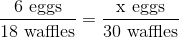For the sake of making math easier, drop the words so we get the following equation: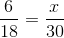To solve for x, cross-multiply this equation.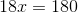Divide both sides by 18.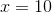### Example Question #2 : Linear Equations With Ratios And Rates

The ratio of boys to girls in a class is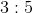. If there areboys in the class, how many girls are in the class?Explanation:

We can write the following equation using the ratios given: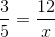is the number of girls in the class.

Now, we can cross multiply to get the following equation: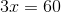Now divide both sides by.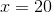### Example Question #2 : Linear Equations With Ratios And Rates

Jessica fills an aquarium with water. The aquarium can hold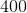gallons of water—it's a huge aquarium! The faucet fills the tank at a rate of 1 gallon every 10 seconds. How long (in seconds) will it take Jessica to fill the tank?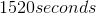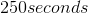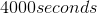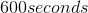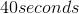Explanation:

The rate at which Jessica fills the tank can be written as a ratio: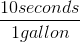Multiply this ratio by the total amount of gallons possible to get the amount of time it takes to fill the tank.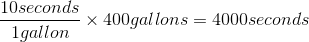Gallon units cancel out, so you are left with seconds as the final unit in the answer.

### All Basic Arithmetic Resources